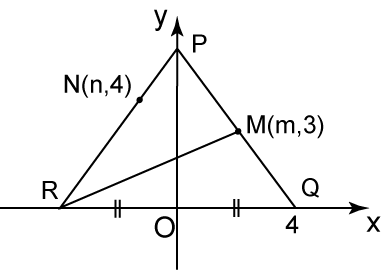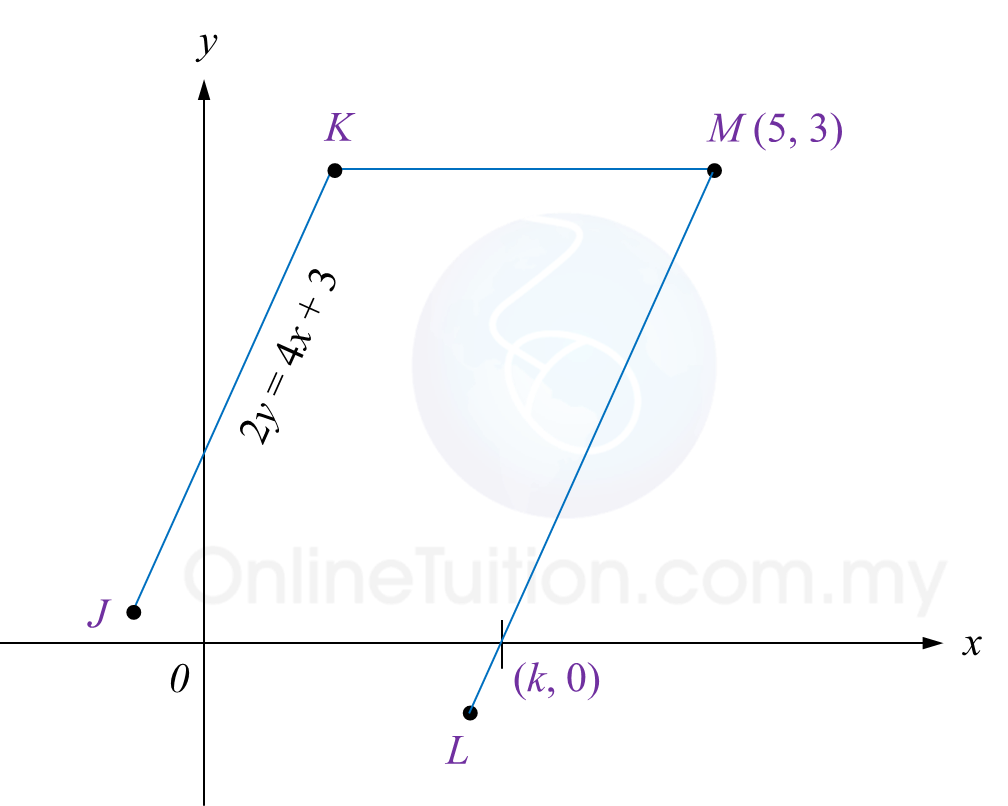# 9.3.5 Straight Lines, PT3 Focus Practice

Question 9:The diagram above shows that the straight line PMQ intersects with PNR at N. Given that OQ = OR and M is the midpoint of PQ. Find
(a) the coordinate of P
(b) the value of m and n.

Solution:

Question 10:
Diagram 10 shows two parallel straight lines, JK and LM, drawn on a Cartesian plane.
The straight line KM is parallel to the x-axis.Diagram 10

Find
(a) the equation of the straight line KM,
(b) the equation of the straight line LM,
(c) the value of k.

Solution:
(a)

The equation of the straight line KM is y = 3

(b)

(c)